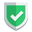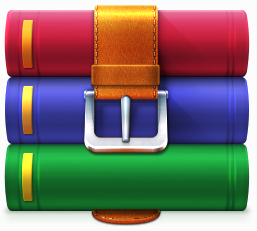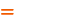我们相信：世界是美好的，你是我也是。平行空间的世界里面，不同版本的生活也在继续...

## `QL\Dom\Elements`

• [HTML属性] 快捷获取html属性
• map() 元素遍历
• attr() 获取html属性
• html() 获取元素的html内容
• text() 获取元素的text内容
• attrs() 获取多个元素的html属性
• htmls() 获取多个元素的html内容
• texts() 获取多个元素的text内容
• children() 获取孩子节点

## 试验演示`demo`

``````\$html = <<<STR
<div id="one">
<div class="two">
<a href="http://querylist.cc">QueryList官网</a>
<img src="http://querylist.com/1.jpg" alt="这是图片" abc="这是一个自定义属性">
<img src="http://querylist.com/2.jpg" alt="这是图片2">
</div>
<span>其它的<b>一些</b>文本</span>
</div>
STR;
\$ql = QueryList::html(\$html);``````

### 快速获取属性

``````//获取第一张图片的链接地址
\$ql->find('img')->src;
//等价下面这句话
\$ql->find('img:eq(0)')->src;
//也等价下面这句话
\$ql->find('img')->eq(0)->src;
//获取第一张图片的abc属性
\$ql->find('img')->abc;
//获取第二张图片的alt属性
\$ql->find('img')->eq(1)->alt;
//等价下面这句话
\$ql->find('img:eq(1)')->alt;``````

### map() 元素遍历

``````\$data = \$ql->find('img')->map(function(\$item){
return \$item->alt;
});
print_r(\$data->all());``````

``````Array
(
 => 这是图片
 => 这是图片2
)``````

### attr() 获取html属性

``````//获取第一张图片的链接地址
\$ql->find('img')->attr('src');
//获取第一张图片的abc属性
\$ql->find('img')->attr('abc');
//获取class为two的元素下的第一个a标签的href属性
\$ql->find('.two>a')->attr('href');``````

### attrs() 获取多个元素的html属性

``````//获取所有的图片链接
\$data = \$ql->find('img')->attrs('src');
print_r(\$data->all());``````

``````Array
(
 => http://querylist.com/1.jpg
 => http://querylist.com/2.jpg
)``````

### htmls() 获取多个元素的html内容

``````\$data = \$ql->find('#one')->append('<div>追加内容</div>')->find('div')->htmls();
print_r(\$data->all());``````

``````Array
(
 => <a href="http://querylist.cc">QueryList官网</a>
<img src="http://querylist.com/1.jpg" alt="这是图片" abc="这是一个自定义属性"><img src="http://querylist.com/2.jpg" alt="这是图片2">
 => 追加内容
)``````

### children() 获取孩子节点

``````//获取class为two元素下的所有img孩子节点
\$data = \$ql->find('.two')->children('img')->attrs('alt');
print_r(\$data->all());
//获取class为two元素下的所有孩子节点
\$data = \$ql->find('.two')->children()->map(function (\$item){
//用is判断节点类型
if(\$item->is('a')){
return \$item->text();
}elseif(\$item->is('img'))
{
return \$item->alt;
}
});
print_r(\$data->all());``````

``````Array
(
 => 这是图片
 => 这是图片2
)
Array
(
 => QueryList官网
 => 这是图片
 => 这是图片2
)``````

## 总结

`elements` 其实就是 `phpquery`对象，那么更多的方法也是很多的。这里不做介绍。请关注苏南大叔的更多`querylist`文章吧。如果本文对您有帮助，或者节约了您的时间，欢迎打赏瓶饮料，建立下友谊关系。本博客不欢迎：各种镜像采集行为。请尊重原创文章内容，转载请保留作者链接。【福利】 腾讯云最新爆款活动！1核2G云服务器首年50元！【源码】本文代码片段及相关软件，请点此获取更多信息【绝密】秘籍文章入口，仅传授于有缘之人querylist